Theory of Probability

1) The mathematical theory of probability assumes that we have a well defined repeatable (in principle) experiment, which has as its outcome a set of well defined, mutually exclusive, events.

Examples:

In the experiment of flipping a coin, the mutually exclusive outcomes are the coin landing either heads up or tails up.

In the experiment of rolling one die, the mutually exclusive outcomes are the die landing with either the 1, 2, 3, 4, 5, or 6 face up.

(When we speak of the "probability" that the Buffalo Bills will win the Super Bowl next year, we are using the word "probability" in its colloquial sense, not its mathematical sense. This is because the Buffalo Bills winning the Super Bowl in a given year is not a repeatable experiment.)

2) We assume that in any particular individual trial of the experiment, the outcome for that individual trial cannot be predicted or known before hand - it is controlled by chance. However, when a very large number of independent trials of the experiment are performed, one finds that each possible outcome occurs a well defined fraction of the time. This fraction is called the probability for that particular outcome to occur. The probability for an outcome is always a number between 0 and 1. If the probability is zero, we say that this outcome can never occur. If the probability is one, we say that this outcome always occurs with complete certainty.

Examples:

When we flip a coin a very large number of times, we find that we get half heads, and half tails. We conclude that the probability to flip a head is 1/2, and the probability to flip a tail is 1/2.

When we role a die a very large number of times, we find that we get any given face 1/6 of the time. The probability for the 1 face to appear is therefore 1/6. Similarly the probability for the 2 (3, 4, 5, or 6) face to appear is also 1/6.

What exactly we mean when we say "a very large number of times" we will come to in a little while (is 10 times "large" enough? is 100 times?)

3) The probability for a given outcome might be calculable from some underlying assumptions. Or the probability for an outcome can be determined experimentally by doing many trials.

Example:

When we flip a coin, we assume that there is no major difference between the two sides, and there is no exacting way we perform the flip in order to try and influence the outcome. Because of all the random factors beyond our control that enter the flipping process (force with which the coin is flipped, motion of the air in the room, position of our hand when we catch the coin...) we therefore expect a probability of 1/2 for heads, and 1/2 for tails. Each possible outcome is equally likely. However, if we did a very large number of trial flips, and consistently found heads occurring 3/4 of the time, and tails 1/4 of the time, we would know that our assumption of equally likely outcomes was false - we are dealing with a loaded coin. Performing the experiment is the way to test the assumptions!

Let's now see how these ideas work for some more complicated examples.

1) Consider the experiment of flipping of 4 coins.

Each coin flip has 2 possible outcomes, so the flipping of 4 coins has 2x2x2x2 = 16 possible outcomes. We can enumerate all possible outcomes as follows, where H indicates a head, and T a tail:

HHHH      THHH

HHHT      THHT

HHTH      THTH

HHTT      THTT

HTHH      TTHH

HTHT      TTHT

HTTH      TTTH

HTTT       TTTT

If we assume that each individual coin is equally likely to come up heads or tails, then each of the above 16 outcomes to 4 flips is equally likely. Each occurs a fraction one out of 16 times, or each has a probability of 1/16.

Alternatively, we could argue that the 1st coin has probability 1/2 to come up heads or tails, the 2nd coin has probability 1/2 to come up heads or tails, and so on for the 3rd and 4th coins, so that the probability for any one particular sequence of heads and tails is just (1/2)x(1/2)x(1/2)x(1/2)=(1/16).

Now lets ask: what is the probability that in 4 flips, one gets N heads, where N=0, 1, 2, 3, or 4. We can get this just by counting the number of outcomes above which have the desired number of heads, and dividing by the total number of possible outcomes, 16.

0                1                                       1/16 = 0.0625

1                4                                       4/16 = 1/4 = 0.25

2                6                                      6/16 = 3/8 = 0.375

3                4                                      4/16 = 1/4 = 0.25

4                1                                      1/16 = 0.0625

We can plot these results on a graph as shown below.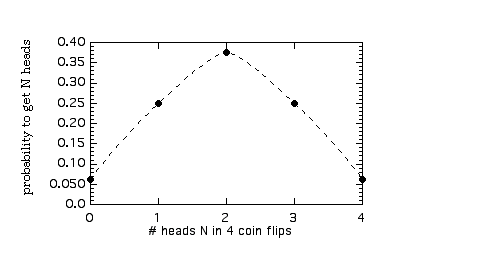The dashed line is shown just as a guide to the eye. Notice that the curve has a "bell" shape. The most likely outcome is for N=2 heads, where the curve reaches its maximum value. This is just what you would expect: if each coin is equally likely to land heads as tails, in four flips, half should come up heads, that is N = 4x(1/2) = 2 is the most likely outcome. Note however that an occurrence of N = 1 or N = 3 is not so unlikely - they occur 1/4 or 25% of the time. To have an occurrence of only N = 0, or N = 4 (no heads, or all heads) is much less likely - they occur only 1/16 or 6.25% of the time.

The above procedure is in principle the way to solve all problems in probability. Define the experiment, enumerate all possible mutually exclusive outcomes (which are usually assumed to be each equally likely), and then count the number of these outcomes which have the particular property being tested for (here for example, the number of heads). Dividing this number by the total number of possible outcomes then gives the probability of the system to have that particular property.

Often, however, the number of possible outcomes may be so large that an explicit enumeration would become very tedious. In such cases, one can resort to more subtle thinking to arrive at the desired probabilities. For example, we can deduce the probabilities to get N heads in 4 flips as follows:

N=0: There is only one possible outcome that gives 0 heads, namely when each flip results in a tail. The probability is therefore 1/16.

N=4: There is only one possible outcome that gives 4 heads, namely when each flip results in a head. The probability is therefore 1/16.

N=1: There are 4 possible outcomes which will have only one coin heads. It may be that the 1st coin is heads, and all others are tails; or it may be that the 2nd coin is heads, and all others are tails; or it may be that the 3rd (or the 4th) coin is heads, and all others are tails. Since there are 4 possible outcomes with one head only, the probability is 4/16 = 1/4.

N=3: To get 3 heads, means that one gets only one tail. This tail can be either the 1st coin, the 2nd coin, the 3rd, or the 4th coin. Thus there are only 4 outcomes which have three heads. The probability is 4/16 = 1/4.

N=2: To enumerate directly all the possible outcomes which have exactly 2 heads only, is a bit trickier than the other cases. We will come to it shortly. But we can get the desired probability for N=2 the following way: We have already enumerated all possible outcomes with either N = 0, 1, 3, or 4 heads. These account for 1 + 4 + 4 + 1 = 10 possible outcomes. The only outcomes not include in these 10 are those with exactly N=2 heads. Since there are 16 possible outcomes, and 10 do not have N=2 heads, there must therefore be exactly 16 - 10 = 6 outcomes which do have exactly N=2 heads. The probability for N=2 is therefore 6/16 = 3/8.

2) Consider the experiment of rolling 3 dice, each of which has 6 sides.

What is the probability that no two dice land with the same number side up, i.e. each of the three dice rolls a different number?

Since each die has 6 possible outcomes, the number of possible outcomes for the roll of three dice is 6x6x6 = 216. We could enumerate all these 216 possibilities, and then count the number of outcomes in which each die has a different number. This is clearly too tedious! Instead we reason as follows:

When the 1st die is rolled, it can land with anyone of its 6 faces up. When the 2nd die is rolled, there are only 5 possible sides which will be consistent with our criterion that no two die land the same. When the 3rd die is rolled, it must land with a face different from die one and two; there are 4 possibilities. Therefore the total number of outcomes which have each of the three dice with a different side up is 6x5x4 = 120.

The probability for this to happen is then 120/216 = 5/9 = .55555...

Alternatively, we can say that the probability for the 1st die to land with any side up is 1, the probability for the 2nd die to land with a side different from the 1st die is 5/6, and the probability for the 3rd die to land with a side different from both the 1st and the 2nd die is 4/6. The probability that each of the three dice has a different side up is then (1)x(5/6)x(4/6) = 20/36 = 5/9.

We learn from the above examples three general rules about probabilities:

1) The probability than any one of a given group of mutually exclusive possible outcomes will actually occur in a given experiment, is the sum of the individual probabilities for each outcome in the group.

Example:

In counting the number of heads in 4 coin flips, the probability that we get exactly one head is the probability that we get anyone of the following 4 outcomes: HTTT, THTT, TTHT, or TTTH. Each has probability 1/16, so the probability to get exactly one head in 4 flips is 1/16 + 1/16 + 1/16 + 1/16 = 4/16 = 1/4. A consequence of this fact is that the sum of the probabilities for all the possible outcomes must equal 1. This is just saying that the probability that the experiment yields some outcome (we don't care which outcome) is just unity, i.e.. it is certain to happen!

2) The probability for two independent events to both occur is just the product of the probabilities for each individual event.

Example:

The probability for no heads to occur in four flips was (1/2)x(1/2)x(1/2)x(1/2) = 1/16. 3) The probability for two events to both occur, even if they are not independent, is the probability for the first to occur, times the probability for the second to occur given the condition that the first has already occurred.

Example:

The probability that the second die lands with a different face than the first is the probability that the 1st lands with any face times the probability that the second lands with a different face, 1x(5/6)=5/6. The probability that the 3rd die lands with yet a different face is 5/6 times the probability that the 3rd die lands with one of the 4 other faces, (5/6)x(4/6) = 20/36 = 5/9. Some more examples:

1) If we roll 4 dice, what is the probability that at least one of them lands with the "6" face on top?

Since each dice can land 6 ways, and there are 4 dice, there are 6x6x6x6 = 64 = 1,296 different possible outcomes. We could in principle write these all down and then count the number which have at least one "6", but this is way too tedious. Instead, lets count the number of outcomes which do not have any "6"s showing. To have no "6" showing, the first dice can land in any one of 5 possible ways. Similarly for the 2nd, 3rd, and 4th die. Thus the number of outcomes with no "6" showing on any die is 5x5x5x5 = 54 = 625. Since we must either see no "6"s, or else we see at least one "6", it follows that the number of outcomes with at least one "6" must be the (total number of outcomes) - (number of outcomes with no "6") = 1,296 - 625 = 671. The probability to have at least one "6" is therefore 671/1296 = 0.5177469...

We could also have arranged our calculation as follows: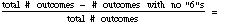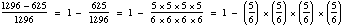Written this way, we see that (5/6) is the probability that any give die lands without a "6" on top. (5/6)x(5/6)x(5/6)x(5/6) is therefore (general rule #2) the probability that all 4 dice land without a "6" on top. Since the probability of all possible outcomes must sum to unity (general rule #1), we therefore conclude that 1 - (5/6)x(5/6)x(5/6)x(5/6) is the probability that the opposite of "all 4 dice land without a "6" on top" happens. But the opposite of "all 4 dice land without a "6" on top" is that "at least one die lands with a "6" on top"!

In this example we see that we can divide all our possible outcomes into two classes. One class which has the desired property we are testing for (in this case, at least one "6" on top), and a second class which does not have the desired property (no dice has a "6" on top). Often it turns out to be hard to directly calculate the probability for the desired property, but easy to calculate the probability not to find the desired property. The first probability is then just unity minus the second probability.

2) What is the probability that in a group of N people, we will find at least 2 people with the same birthday? For the purpose of this question, we will consider people born on February 29 as if they were born on February 28!

This is equal to 1 - (probability no 2 people have same birthday)

Probability that no 2 people have the same birthday is: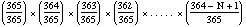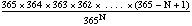since the first person can have any birthday, the second person can have only one of the remaining 364 out of 365 possible birthdays (i.e. not the same as the first), the third person can have only one of the remaining 363 out 365 possible birthdays (i.e. not the same as either the first or the second), and so on, down to the last Nth person. So the probability that at least 2 people in a group of N have the same birthday is:

p(N) = 1 -We can plot p(N) versus N on a graph, as shown below.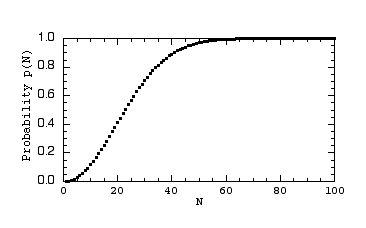Note that for N=10, p(10)=0.11695, or about 12% chance to have two people with the same birthday. For N=50, p(50)=0.97037, or 97% chance to have two people with the same birthday. These probabilities are in general much larger than most people's intuition would have guessed.

Examples from Text:

1) Two fair dice are rolled. What is the probability p that at least one die come up a 3?

We can do this two ways:

i) The straightforward way is as follows. To get at least one 3, would be consistent with the following three mutually exclusive outcomes:

the 1st die is a 3 and the 2nd is not: prob = (1/6)x(5/6)=5/36

the 1st die is not a 3 and the 2nd is: prob = (5/6)x((1/6)=5/36

both the 1st and 2nd come up 3: prob = (1/6)x(1/6)=1/36

sum of the above three cases is prob for at least one 3, p = 11/36

ii) A faster way is as follows: prob at least one 3 = 1 - (prob no 3's)

The probability to get no 3's is (5/6)x(5/6) = 25/36.

So the probability to get at least one 3 is, p = 1 - (25/36) = 11/36

2) What is the probability that a card drawn at random from an ordinary 52 deck of playing cards is a queen or a heart?

There are 4 queens and 13 hearts, so the probability to draw a queen is

4/52 and the probability to draw a heart is 13/52. But the probability to draw a queen or a heart is NOT the sum 4/52 + 13/52. This is because drawing a queen and drawing a heart are not mutually exclusive outcomes - the queen of hearts can meet both criteria! The number of cards which meet the criteria of being either a queen or a heart is only 16 - the 4 queens and the 12 remaining hearts which are not a queen. So the probability to draw a queen or a heart is 16/52 = 4/13.

3) Five coins are tossed. What is the probability that the number of heads exceeds the number of tails?

We can divide all possible outcomes into the following two mutually exclusive groups:

i) the number of heads flipped is more than the number of tails flipped

ii) the number of tails flipped is more than the number of heads flipped

Since the probability to flip a head is the same as the probability to flip a tail, the probability of outcome (i) must be equal to the probability of outcome (ii). So both must be equal to 1/2.

Note that this answer works for any odd number of coin flips.

4) 4 boys and 3 girls are standing in a line. If the position of each child in line is random, what is the probability that the first 3 places in line are all girls?

We will solve this problem two ways:

i) Lets us count the number of all the possible orderings of the 7 children which are consistent with the desired outcome (i.e. 3 girls in front) and divide by the total number of all possible orderings.

The total number of orderings is: 7x6x5x4x3x2x1

as there are 7 children to pick to be 1st in line, only 6 remaining children to pick to be 2nd in line, then only 5 remaining children to pick to be 3rd in line, and so on.

The number of orderings consistent with 3 girls in front is: (3x2x1)x(4x3x2x1)

as there are 3 girls to pick to be 1st in line, 2 remaining girls to pick to be 2nd in line, and the last girl must go 3rd in line; then there are 4 boys to place 4th in line, 3 remaining boys to place 5th in line, and so on.

So the probability to get all 3 girls in front is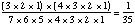ii) Alternatively, we can argue as follows. The probability that a girl is 1st in line is (3/7) since 3 of the 7 children are girls. The probability that a girl is 2nd in line, given that the 1st is a girl, is (2/6) since 2 of the remaining 6 children are girls. The probability that a girl is 3rd in line, given that the 1st and 2nd are girls, is (1/5) since now only 1 of the remaining 5 children is a girl. So the probability that the 1st, 2nd, and 3rd positions are all girls is

(3/7)x(2/6)x(1/5)=1/35